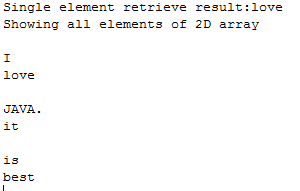# 2D Array in JAVA

## 2D arrays in JAVA

In this part of tutorial you will learn 1) What is a 2D array? 2) Why we use 2D arrays? 3) How we use 2D arrays in java programming? What is a 2D array? One dimensional array is a linear array, while the two dimensional array is such an array which has the rows as well as columns. The 2 dimensional is an array within an array so the each entry is divided further allowing us more entries to be included. It is a nonlinear array which is has a vertical length and horizontal length as well. Why we use 2D arrays? To visualize certain real life things in programming terms two dimensional arrays are used. The chess game and digital image can be represented using the two dimensional arrays. The image has some value at each of its pixel, similarly the chess game has values present at each point. One dimensional and two dimensional arrays are just the same but they only differ in their representation as if we want to place the course being taught in a list form or in the form of table, its upon our choice, so we may use the 2D array or 1D array. The two dimensional array can be thought of a matrix for our ease of understanding. How we use the 2D arrays in JAVA? The two dimensional arrays have the properties same as that of the one dimensional arrays. The insertion and deletion can be done at any point. The syntax for declaring and initializing an array is same in both. The syntax is
`String[][] my2Darray = {{ "I",  "love" },				{ "JAVA", "it" },				{ "is",  "best"  }};`
To access an element of the array we use the same method as of the one dimensional array but the rows as well as columns have to be mentioned. The first entry represent the row and the second entry represent the columns. We can also use a for loop to retrieve the elements of a 2D array. As shown below
`for (int i=0; i< col ; i++)for (int j = 0; j < rows; j++) {			   System.out.print(my2Darray[i][j]);			   System.out.println();			  }`
Deletion We can also delete the entries of an array by assigning them zero value. As for the above array the data type of the 2D array is string so we set a value equal to null for each element using the for loop.
`for (int i = 0; i <3; i++) {			System.out.println();			   for (int j = 0; j < 2; j++) {			  my2Darray[i][j]=null; 			  }		}`
Code Example: This program has been written to clear your concepts about the 2D arrays in java.
`package test;import java.util.*;public class myclass { 	public static void main(String[] args) {		String[][] my2Darray = {{ "I",  "love" },				{ "JAVA.", "it" },				{ "is",  "best"  }};  		System.out.print("Single element retrieve result:");	System.out.print(my2Darray); // element wise data extraction	System.out.println();	System.out.print("Showing all elements of 2D array");	System.out.println(); 		for (int i = 0; i <3; i++) {   //used to extract whole contact of 2D array			System.out.println();			   for (int j = 0; j < 2; j++) {			   System.out.print(my2Darray[i][j]);			   System.out.println();			  }			}		//the code below is for deletion of all the elements of the array		/*for (int i = 0; i <3; i++) { 			System.out.println();			   for (int j = 0; j < 2; j++) {			  my2Darray[i][j]=null;			  System.out.println();			  }		} */	} }`
ScreenShot